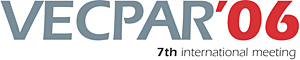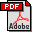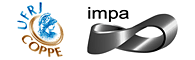vecpar.fe.up.pt/2006 | vecpar2006@fe.up.pt Simulation of Laser Propagation With a Frequency Maxwell Equation Remi Sentis (CEA/Bruyeres)Sylvian S.\ Desroziers (CEA/Bruyeres)Frederic Nataf (University Paris VI) Abstract: The aim of this work is to perform numerical simulations of the propagation of a laser beam in a plasma. At each time step, one has to solve a Helmholtz equation with variable coefficients in a domain which may contain more than hundred millions of cells. One uses an iterative method of Krylov type to deal with this system. At each inner iteration, the preconditioning amounts essentially to solve a linear system which corresponds to the same five-diagonal symmetric non-hermitian matrix. If \$n_{x}\$ and \$n_{y}\$ denote the number of discretization points in each spatial direction, this matrix is block tri-diagonal and the diagonal blocks are equal to a square matrix \$A\$ of dimension \$n_{x}\$ which corresponds to the discretization form of a one-dimension wave operator. The corresponding linear system is solved by a block cyclic reduction method. The crucial point is the product of a full square matrix \$Q\$ of dimension \$% n_{x}\$ by a set of \$n_{y}\$ vectors where \$Q\$ corresponds to the basis of the \$n_{x}\$ eigenvectors of the tri-diagonal symmetric matrix \$A\$. We show some results which are obtained on a parallel architecture. Simulations with 200 millions of cells have run on 200 processors and the results are presented. Keywords: Numerical Methods (Linear algebra)Download the fullpaper TopRio de Janeiro | Brazil | 2006 | July | 10 11 12 13